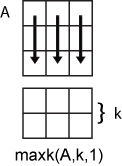# maxk

## 语法

``B = maxk(A,k)``
``B = maxk(A,k,dim)``
``B = maxk(___,'ComparisonMethod',c)``
``[B,I] = maxk(___)``

## 说明

````B = maxk(A,k)` 返回 `A` 的 `k` 个最大元素。 如果 `A` 是向量，则 `maxk` 返回一个向量，其中包含 `A` 的 `k` 个最大元素。如果 `A` 是矩阵，则 `maxk` 返回一个矩阵，此矩阵的列中包含 `A` 的每一列中的 `k` 个最大元素。如果 `A` 是多维数组，则 `maxk` 返回大小不等于 1 的第一个维度上的 `k` 个最大元素。 ```

``B = maxk(A,k,dim)` 确定 `A` 在维度 `dim` 上的 `k` 个最大元素。`

``B = maxk(___,'ComparisonMethod',c)` 为上述任何语法指定如何比较 `A` 的元素，此项为可选项。例如，`maxk(A,k,'ComparisonMethod','abs')` 根据绝对值返回 `A` 的 `k` 个最大元素。`

``[B,I] = maxk(___)` 计算 `A` 的 `k` 个最大值的索引，并在 `I` 中返回这些索引。`

## 示例

```A = 1:10; B = maxk(A,3)```
```B = 1×3 10 9 8 ```

`A = magic(5)`
```A = 5×5 17 24 1 8 15 23 5 7 14 16 4 6 13 20 22 10 12 19 21 3 11 18 25 2 9 ```
`B = maxk(A,3,2)`
```B = 5×3 24 17 15 23 16 14 22 20 13 21 19 12 25 18 11 ```

`A = [2-2i 5+i -7-3i -1+i]`
```A = 1×4 complex 2.0000 - 2.0000i 5.0000 + 1.0000i -7.0000 - 3.0000i -1.0000 + 1.0000i ```
`[B,I] = maxk(A,2,'ComparisonMethod','abs')`
```B = 1×2 complex -7.0000 - 3.0000i 5.0000 + 1.0000i ```
```I = 1×2 3 2 ```

## 输入参数

• 如果 `A` 是向量，则 `maxk` 返回一个向量，其中包含 `A``k` 个最大元素。

• 如果 `A` 是矩阵，则 `maxk` 返回一个矩阵，此矩阵的列中包含 `A` 的每一列中的 `k` 个最大元素。

• 如果 `A` 是多维数组，则 `maxk` 返回大小不等于 1 的第一个维度上的 `k` 个最大元素。`maxk(A,k,2)` 沿 `A` 的列进行运算，计算每一行的最大值。• `'auto'` - 当 `A` 为实数时，按 `real(A)` 对输入 `A` 的元素进行比较；当 `A` 为复数时，按 `abs(A)` 进行比较。

• `'real'` - 当 `A` 为实数或复数时，按 `real(A)` 对输入 `A` 的元素进行比较。如果 `A` 包含具有相等实部的元素，则使用 `imag(A)` 进行排序。

• `'abs'` - 当 `A` 为实数或复数时，按 `abs(A)` 对输入 `A` 的元素进行比较。如果 `A` 包含具有相等模的元素，则使用区间 (-π,π] 中的 `angle(A)` 进行排序。